# 11 2 Volume of Prisms and Cylinders Objectives

• Slides: 9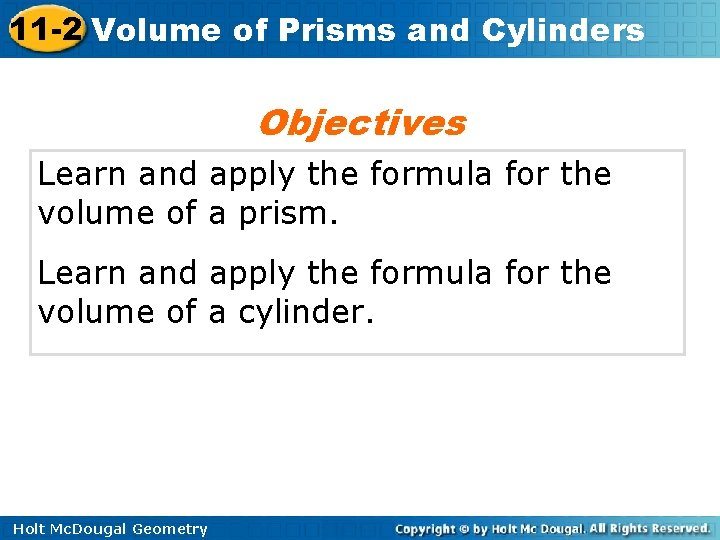11 -2 Volume of Prisms and Cylinders Objectives Learn and apply the formula for the volume of a prism. Learn and apply the formula for the volume of a cylinder. Holt Mc. Dougal Geometry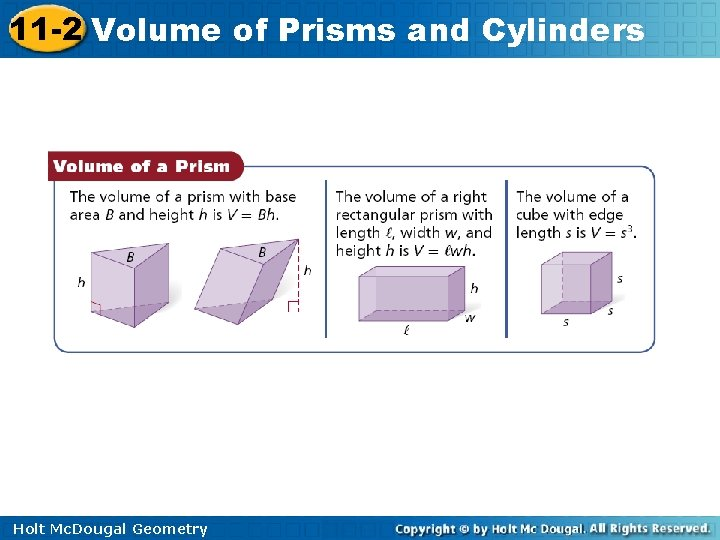11 -2 Volume of Prisms and Cylinders Holt Mc. Dougal Geometry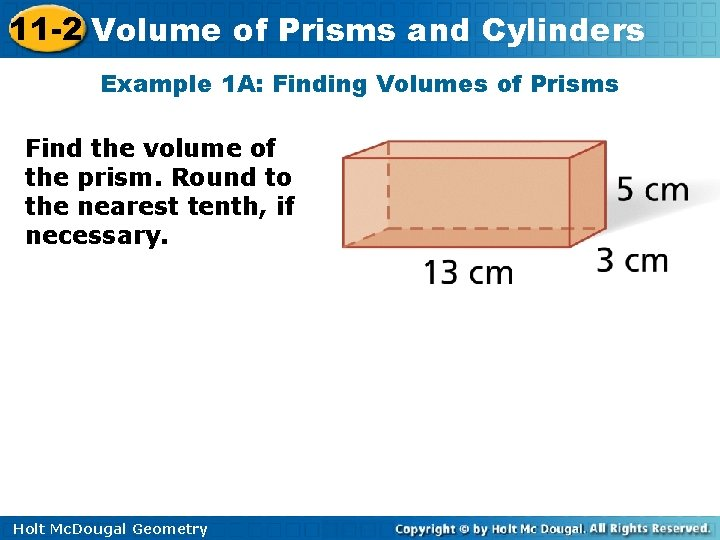11 -2 Volume of Prisms and Cylinders Example 1 A: Finding Volumes of Prisms Find the volume of the prism. Round to the nearest tenth, if necessary. Holt Mc. Dougal Geometry11 -2 Volume of Prisms and Cylinders Example 1 B: Finding Volumes of Prisms Find the volume of a cube with edge length 15 in. Round to the nearest tenth, if necessary. Holt Mc. Dougal Geometry11 -2 Volume of Prisms and Cylinders Check It Out! Example 1 c Find the volume of a triangular prism with a height of 9 yd whose base is a right triangle with legs 7 yd and 5 yd long. Holt Mc. Dougal Geometry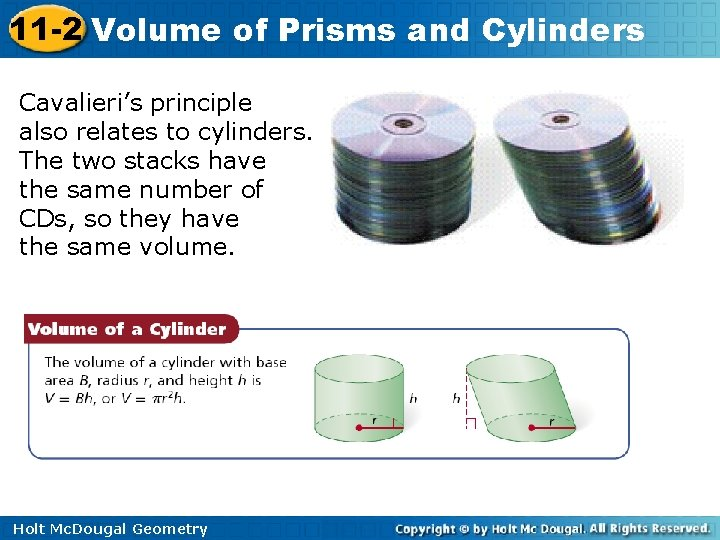11 -2 Volume of Prisms and Cylinders Cavalieri’s principle also relates to cylinders. The two stacks have the same number of CDs, so they have the same volume. Holt Mc. Dougal Geometry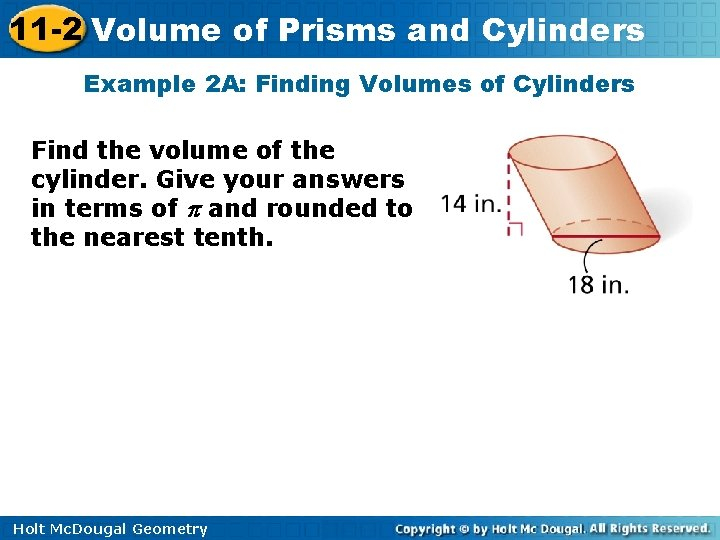11 -2 Volume of Prisms and Cylinders Example 2 A: Finding Volumes of Cylinders Find the volume of the cylinder. Give your answers in terms of and rounded to the nearest tenth. Holt Mc. Dougal Geometry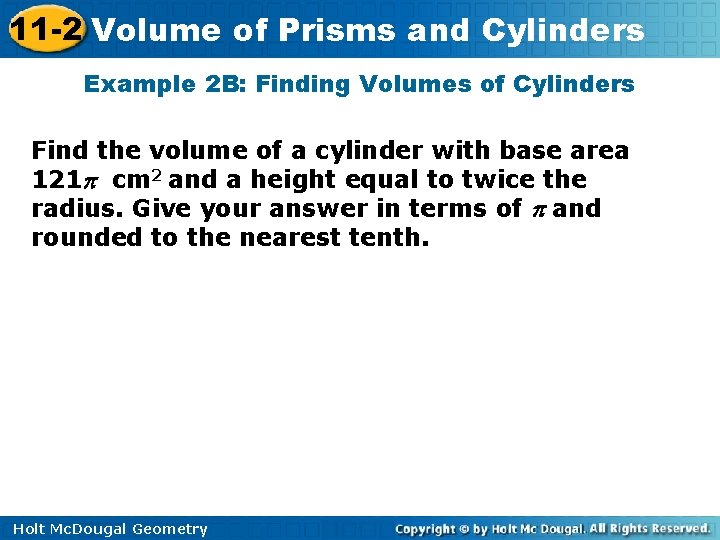11 -2 Volume of Prisms and Cylinders Example 2 B: Finding Volumes of Cylinders Find the volume of a cylinder with base area 121 cm 2 and a height equal to twice the radius. Give your answer in terms of and rounded to the nearest tenth. Holt Mc. Dougal Geometry11 -2 Volume of Prisms and Cylinders Check It Out! Example 2 c Find the volume of a cylinder with a diameter of 16 in. and a height of 17 in. Give your answer both in terms of π and rounded to the nearest tenth. Holt Mc. Dougal Geometry